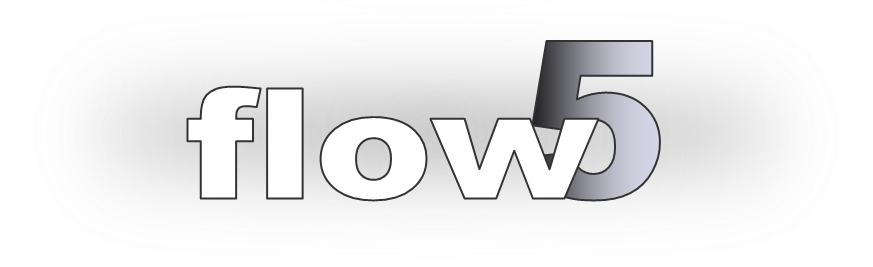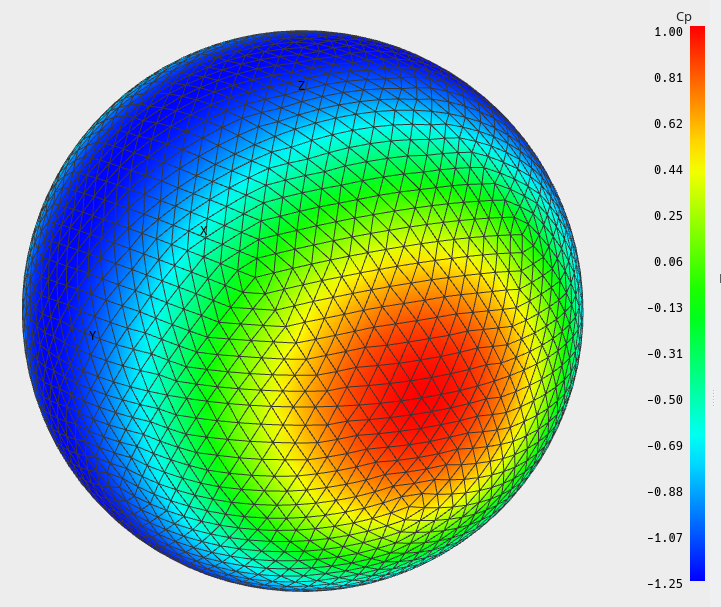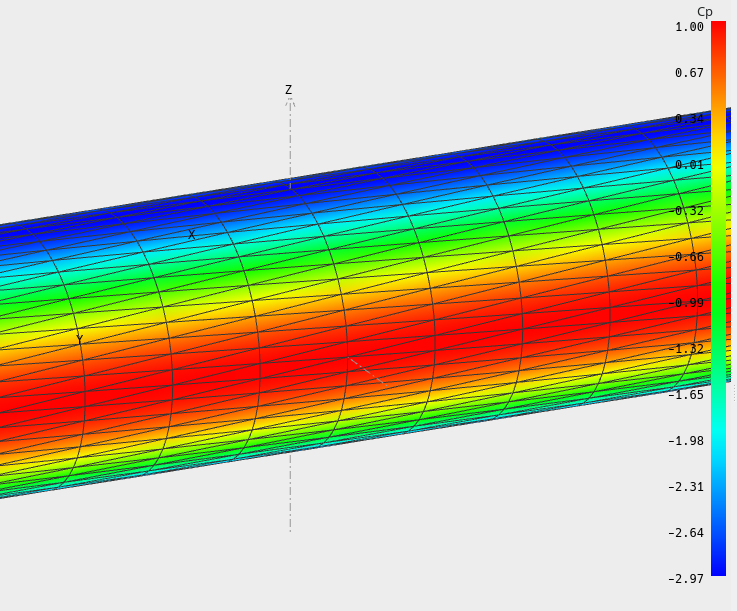# Validation of the triangle Galerkin method

Updated June 7th, 2020

## Contents

The following results were obtained after improvement of the triangular linear method in V7.01 beta 09.

## Sphere

The pressure coefficient on the surface of a sphere in the plane of symmetry is Cp = 1 - 9/4 sin²(θ)
where the origin of the angle θ is taken at the leading point.
The pressure coefficients are therefore:
Cp=-1.25 at the top point.The error decreases with the number of surface elements:It can be noted that the linear method is more precise, but that the uniform methods catches up in precision for mesh sizes greater than 1000.

## Cylinder

The pressure coefficient on the surface of a cylinder is Cp = 2 cos(2θ)-1
where the origin of the angle θ is taken at the leading point.
The pressure coefficients are therefore:
Cp=-3 at the top point.